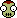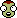## Recommended Posts

My texture is not loading in scaled space. Here is my config:

```@Kopernicus:AFTER[KOPERNICUS]
{
Body
{
name = Nok
Template
{
name = Eeloo
removeAllPQSMods = true
}
Properties
{
despcription = Nok is a frozen planet at the edge of the Kerbol System. The scientist who discovered it beleived it was a snowball being thrown at him and promptly hid behind a desk. As a result of this incident, the astronomical community has voted to sentence Nok to 100 years in prison, although no arrest has yet been made.
geeASL = 0.20
rotationPeriod = 45000
rotates = true
tidallyLocked = false
initialRotation = 0
isHomeWorld = false
timewarpAltitudeLimits = 0 2500 5000 20000 20000 50000 150000 500000
ScienceValues
{
landedDataValue = 7
splashedDataValue = 1
flyingLowDataValue = 6
flyingHighDataValue = 5
inSpaceLowDataValue = 5
inSpaceHighDataValue = 3
recoveryValue = 9
flyingAltitudeThreshold = 5000
spaceAltitudeThreshhold = 200000
}

}
Orbit
{
referenceBody = Sun
color = 1,1,1,0.5
inclination = 9.72
eccentricity = 0.19
semiMajorAxis = 200000000000
longitudeOfAscendingNode = 0
argumentOfPeriapsis = 0
meanAnomalyAtEpoch = 0
epoch = 0
}
ScaledVersion
{
type = Vacuum
Material
{
texture = Nok/PluginData/NokColor.dds
normals = Nok/PluginData/NokNormal.dds
shininess = 0
specular = 0.0,0.0,0.0,1.0
}
}
PQS
{
Mods
{
VertexHeightMap
{
map = Nok/PluginData/NokHeight.dds
offset = 100
deformity = 500
order = 20
enabled = true
}
VertexHeightNoiseVertHeightCurve2
{
deformity = 100
ridgedSubSeed = 654321
ridgedSubFrequency = 12
ridgedSubLacunarity = 2
ridgedSubOctaves = 4
simplexCurve
{
key = 0 0 0.1466263 0.1466263
key = 0.7922793 0.2448772 0.6761706 1.497418
key = 1 1 6.106985 6.106985
}
simplexHeightStart = 0
simplexHeightEnd = 6500
simplexSeed = 123456
simplexOctaves = 4
simplexPersistence = 0.6
simplexFrequency = 12
enabled = true
order = 200
}
HeightColorMap
{
blend = 1
order = 500
enabled = true
LandClasses
{
Class
{
name = Bottom
altitudeStart = 0
altitudeEnd = 0.7
color = 0.1,0.1,0.1,1.0
lerpToNext = true
}
Class
{
name = Base
altitudeStart = 0.7
altitudeEnd = 0.75
color = 0.7,0.55,0.2,1.0
lerpToNext = true
}
Class
{
name = Low
altitudeStart = 0.75
altitudeEnd = 0.85
color = 0.7,0.6,0.4,1.0
lerpToNext = true
}
Class
{
altitudeStart = 0.85
altitudeEnd = 0.95
color = 1.0,0.9,0.7,1.0
lerpToNext = true
}
Class
{
name = High
altitudeStart = 0.95
altitudeEnd = 2
color = 0.95,0.95,0.9,1.0
lerpToNext = false
}
}
}
}
}
}
}```

Edited by KingKerb

##### Share on other sites
On 1/20/2020 at 11:21 AM, KingKerb said:

My texture is not loading in scaled space. Here is my config:

```
@Kopernicus:AFTER[KOPERNICUS]
{
Body
{
name = Nok
Template
{
name = Eeloo
removeAllPQSMods = true
}
Properties
{
despcription = Nok is a frozen planet at the edge of the Kerbol System. The scientist who discovered it beleived it was a snowball being thrown at him and promptly hid behind a desk. As a result of this incident, the astronomical community has voted to sentence Nok to 100 years in prison, although no arrest has yet been made.
geeASL = 0.20
rotationPeriod = 45000
rotates = true
tidallyLocked = false
initialRotation = 0
isHomeWorld = false
timewarpAltitudeLimits = 0 2500 5000 20000 20000 50000 150000 500000
ScienceValues
{
landedDataValue = 7
splashedDataValue = 1
flyingLowDataValue = 6
flyingHighDataValue = 5
inSpaceLowDataValue = 5
inSpaceHighDataValue = 3
recoveryValue = 9
flyingAltitudeThreshold = 5000
spaceAltitudeThreshhold = 200000
}

}
Orbit
{
referenceBody = Sun
color = 1,1,1,0.5
inclination = 9.72
eccentricity = 0.19
semiMajorAxis = 200000000000
longitudeOfAscendingNode = 0
argumentOfPeriapsis = 0
meanAnomalyAtEpoch = 0
epoch = 0
}
ScaledVersion
{
type = Vacuum
Material
{
texture = Nok/PluginData/NokColor.dds
normals = Nok/PluginData/NokNormal.dds
shininess = 0
specular = 0.0,0.0,0.0,1.0
}
}
PQS
{
Mods
{
VertexHeightMap
{
map = Nok/PluginData/NokHeight.dds
offset = 100
deformity = 500
order = 20
enabled = true
}
VertexHeightNoiseVertHeightCurve2
{
deformity = 100
ridgedSubSeed = 654321
ridgedSubFrequency = 12
ridgedSubLacunarity = 2
ridgedSubOctaves = 4
simplexCurve
{
key = 0 0 0.1466263 0.1466263
key = 0.7922793 0.2448772 0.6761706 1.497418
key = 1 1 6.106985 6.106985
}
simplexHeightStart = 0
simplexHeightEnd = 6500
simplexSeed = 123456
simplexOctaves = 4
simplexPersistence = 0.6
simplexFrequency = 12
enabled = true
order = 200
}
HeightColorMap
{
blend = 1
order = 500
enabled = true
LandClasses
{
Class
{
name = Bottom
altitudeStart = 0
altitudeEnd = 0.7
color = 0.1,0.1,0.1,1.0
lerpToNext = true
}
Class
{
name = Base
altitudeStart = 0.7
altitudeEnd = 0.75
color = 0.7,0.55,0.2,1.0
lerpToNext = true
}
Class
{
name = Low
altitudeStart = 0.75
altitudeEnd = 0.85
color = 0.7,0.6,0.4,1.0
lerpToNext = true
}
Class
{
altitudeStart = 0.85
altitudeEnd = 0.95
color = 1.0,0.9,0.7,1.0
lerpToNext = true
}
Class
{
name = High
altitudeStart = 0.95
altitudeEnd = 2
color = 0.95,0.95,0.9,1.0
lerpToNext = false
}
}
}
}
}
}
}```

The most likely culprit is your file paths being wrong, but just to be sure, upload Nok.body.log (found in KSP Root/Logs/Kopernicus, where KSP root is the folder with the game executable in it).

##### Share on other sites

Hello, I'm making a planet/star pack and a semi-transparent blue-ish bar appeared between the day and night side of one of my planets. Please help!Also, how do I use HazardousBody? I am confused.

##### Share on other sites
Posted (edited)

Hello. In the development of my planet pack I stumbled across two errors: first, the ground textures won't show up, second, this:

PLEASE HELP!!!!Sorry for my (kinda) bad English, it's not my native language.

Edited by E for Earthling

##### Share on other sites

How can I modify the Sun's luminosity curve without making my planets' orbits go crazy?

## Join the conversation

You can post now and register later. If you have an account, sign in now to post with your account.
Note: Your post will require moderator approval before it will be visible.